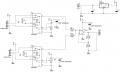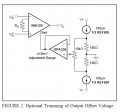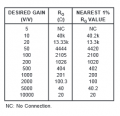# Load cell amplifier and summing circuit

#### vaka85

Joined Aug 21, 2014
40
Hi all,

i'm designing a circuit to amplify two extensometer (Flintec XT50 http://www.flintec.com/Type_XT50_Extensometer.html) and sum their values.
I would like to know if in your opinion it is ok or there is something to change.
The power supply is given by a 12 V battery as input for a LM7805 regulator.

The main issue that I'm experiencing is that the gain is not so high. I cannot use an high gain resistor, since with an input that is very low (some mV), as soon as I go over 1 KOhm for the gain resistor, the output saturates and goes close to 5 V.
There is any kind of configuration for the inputs that I should take into account?
What I'm doing wrong?
Thanks#### GopherT

Joined Nov 23, 2012
8,012
@vaka85

You should be using an op-amp buffer for your Pin 5 reference voltage, not just a voltage divider with resistors. You should also have a capacitor from pin 5 to pin 4 to stablize your reference voltage (virtual ground).

from the TI datasheet...Also, if you have signal of a few millivolts and your amp saturates, you need to calculate what gain you need. Here is the chart from the TI datasheet as well.#### AnalogKid

Joined Aug 1, 2013
8,806
What he said, plus... You don't say which amp is clipping, and it could be all of them. Everything is DC coupled and there is less than 5V of headroom, so it won't take much imbalance somewhere to hit one of the rails. Also, IC4 is not a true summer. If, for example, the outputs of IC1 and IC5 are each 1.0 V, the output of IC4 will be 2.0V (no summing, absolute value times 2), not 4.0V ((1 V + 1 V) x 2).

ak

#### vaka85

Joined Aug 21, 2014
40
@GopherT: sorry for asking, but what's the reason to put an opamp for the reference voltage instead of using resistors?
I was also wondering if the offset voltage is really needed. After some tests I saw that I have only mono-polar output from the load cell, so maybe it's not needed. Is it?

I calculated the right value for the gain, to have an output of about 2 V when the input is very low, but my calculations don't mach the practical results.. I don't know why.

@AnalogKid: IC1 and IC5 have this problem.
I know that IC4 isn't a true summer, I don't want a factor of 2 at the output. So it's correct that if out1=1 and out2=1, total output should be 2V.

Thanks

#### GopherT

Joined Nov 23, 2012
8,012
@GopherT: sorry for asking, but what's the reason to put an opamp for the reference voltage instead of using resistors?
Look at the resistors in the chip. Pin 6 (output) has a resistor network back to the ref pin (pin 5) of 2 x 100k + the gain resistor = 201k ohm in the INA. Now, add that 201k ohm resistance in parallel with your top 100k ohm resistor in your voltage divider. Instead of 100k and 100k to make 2.5V midpoint, you really have (100k || 201k) = 67k.

A voltage divider of 67k and 100k gives a reference voltage of 3.3v when the output is near 5 volts and it swings the other way (Vref = 1.67 volts) when the output on pin6 is near zero volts.

If that is close enough for you, then don't use the op amp.

I was also wondering if the offset voltage is really needed. After some tests I saw that I have only mono-polar output from the load cell, so maybe it's not needed. Is it?
I don't know your needs and range of your measurements and needs for calibration.

#### vaka85

Joined Aug 21, 2014
40
thanks for the explanation.

The voltage you are talking about are quite good for my application, I have to read the value with a microcontroller.
I try to explain better my goal.
I need to calibrate the system to obtain an output swing as bigger as possible. I calibrated the zero kg point at a point near 0 V.
Since my maximum weight to be measured will be about 1000 kg, but it's impossible at the moment to test the system with such an heavy load, I tried with 300 kg. I played with the gain to have a voltage a little less than half the scale, i.e. 2 V, when the extensiometer is holding 300 kg..

In your opinion the procedure is ok?
I can have any voltage at the output, not necessary 0 and 5, since the calibration will be done by the microcontroller. But the output should be big enough to be read by it (The resolution of the microcontroller is 5 mV).

#### AnalogKid

Joined Aug 1, 2013
8,806
"I calculated the right value for the gain, to have an output of about 2 V when the input is very low, but my calculations don't mach the practical results.. I don't know why."

It's because the IC4 circuit is not what you think it is.

"I know that IC4 isn't a true summer, I don't want a factor of 2 at the output. So it's correct that if out1=1 and out2=1, total output should be 2V."

No, it shouldn't. IC4 has a fixed factor (gain) of 2 no matter what its inputs are. It is a gain-of-2 non-inverting amplifier. Try this: If the two sensor circuits are 1.0V and 0.5V, the voltage at IC4 pin3 is 0.75V. This is average value of the combined inputs, not the sum, the difference, the absolute value of the greater, or any other usual result of combining two sensor outputs. After IC4's gain of 2, its output is 1.5 V, and looks like the sum of the two sensor outputs. It isn't, and it can't ever be with the circuit you have drawn. One way to see this is to eliminate R12. This changes IC4 to a voltage follower with a voltage gain of 1 (no "factor"). Now its output will represent the true nature of the combined sensor signals. 1 + 1V will equal 1V, 1V + 0.5V will equal 0.75V, etc.

ak

#### vaka85

Joined Aug 21, 2014
40
Hi @AnalogKid,

sorry but I don't see your point.
If we take two sensor signals, 1 V and 0,5 V. At the output with the current configuration I have 1,5 V.
Why you say that it's not a real sum? It first do the average, and then multiplies by 2. I don't understand what's the difference...

#### ericgibbs

Joined Jan 29, 2010
11,608
hi,
This LTS sim of your summer shows the operation.
Normally the Gain of an non Inverting OPA is Vo = 1 + [Rfb/Rin].

E
EDIT:
This second image shows the Vsum at the Non Inv input

#### vaka85

Joined Aug 21, 2014
40
Hi Eric,
yes, this confirms what I was saying.. doesn't it?
If I'm not correct, what I have to do to combine the weight measured by the two extensiometers?
thanks

Last edited:

#### ericgibbs

Joined Jan 29, 2010
11,608
hi vaka,
Yes, it does.
E
EDIT:
Others have explained that you need a low output impedance source for the Vref voltage to the IA's. It's important to do that if you want accurate results.

#### ericgibbs

Joined Jan 29, 2010
11,608
hi,
The sensor data sheet says 1.2mV/V, what the Vext and amplifier supply voltage.?

#### vaka85

Joined Aug 21, 2014
40
Yes, I already changed the Vref as Gopher suggested. Now it seems to work better.

I also changed the power supply to 12 V, since I already have a stable 12 V power source.
So now the load cell and the amplifier have both 12 V. (even if the loadcell datasheet says from 5 to 10 V, I don't think there will be any problem if I use 12 V... They are only resistors..)
1.2 mV/V means 14.4 mV with 12 V supply. It means that at the calculated maximum strain I will have about 15 mV. If the amplifier gain is 400, I should have 5.6 V at the output.
Is this correct?
If so, the remaining problem is the sum of the 2 weights...

#### ericgibbs

Joined Jan 29, 2010
11,608
hi,
As you say 1.2mV * 12 = 14.4mV per bridge, so one bridge INA122 will give 400 * .0144V =5.76V output,

If you now use a non inverting 'summer' , you will get 5.76Vout from the summer amp, when the two bridges are fully load.
What have you set the Vout to be when the input from the sensors is 0v.? ie: what is your Vref offset.?

E

#### ericgibbs

Joined Jan 29, 2010
11,608
hi,
This is a LTS Sim of your amp.
Using a +/-10mV signal for each bridge and a +3V ref/offset
E.
Have you considered just putting the bridges in parallel and using only one INA122 IA.?

#### vaka85

Joined Aug 21, 2014
40
Eric, thank you for your support!
The signal from the bridge is about 10 mV, the variations are not readable with a multimeter, it's always at 10 mV.
Vref is 5 V. I measured, with 400 kg load, a variation of about 400 mV at the output. I hope that the relationship remains linear even with higher weights..
I don't want to put the two bridges in parallel because I want to amplify the signal close to the cells, and since the cells are not close one to each other, I cannot do it.

I still don't see why no one likes my summing configurationsorry..

#### ericgibbs

Joined Jan 29, 2010
11,608
hi,
With Vref at 5V, the higher range Vout output voltage will clip.!
I would suggest 3Vref.

How do you plan to display the results from the Vout.?
E